maths > differential-calculus

Differentiation: Graphical Meaning

what you'll learn...

Overview

»  Graphical Meaning of Derivative: slope of the tangent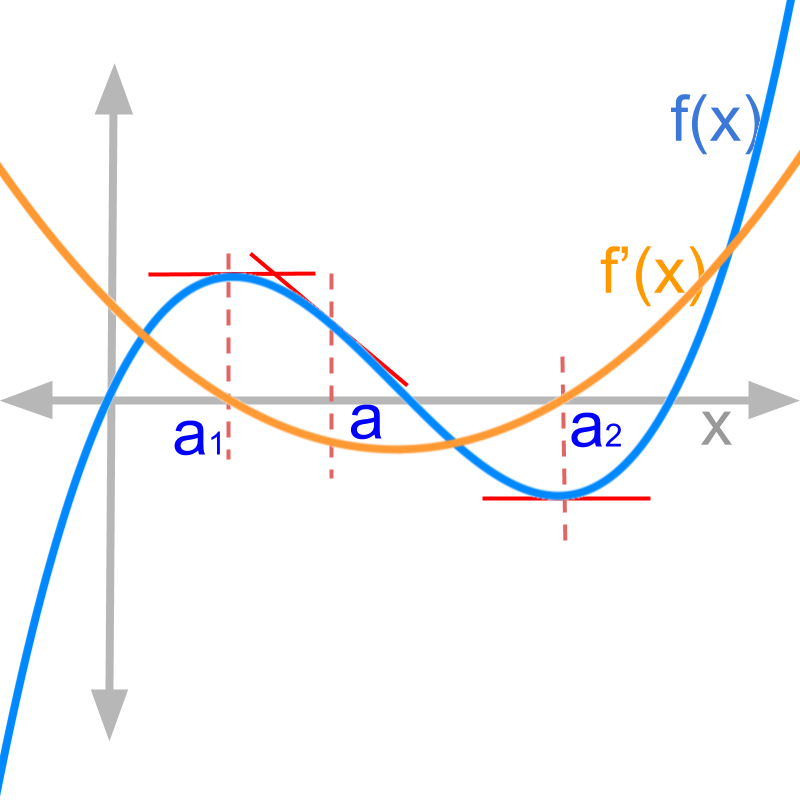→  at a point $x=a$$x = a$, the rate of change of the curve is $f\prime \left(a\right)$

→  at a point $x=a$$x = a$, the slope of the tangent is $f\prime \left(a\right)$.

→  at the maxima of the curve $f\left({a}_{1}\right)$$f \left({a}_{1}\right)$, the derivative $f\prime \left({a}_{1}\right)$ crosses $0$$0$ from positive rate of change to negative rate of change.

→  at the minima of the curve $f\left({a}_{2}\right)$$f \left({a}_{2}\right)$, the derivative $f\prime \left({a}_{2}\right)$ crosses $0$$0$ from negative rate of change to positive rate of change.

recap

Summary of differentiation: Given $y=f\left(x\right)$$y = f \left(x\right)$ a function of variable $x$$x$, the derivative of $y$$y$ is

$\frac{dy}{dx}$$\frac{\mathrm{dy}}{\mathrm{dx}}$$=\underset{\delta \to 0}{lim}\frac{f\left(x+\delta \right)-f\left(x\right)}{\delta }$$= {\lim}_{\delta \to 0} \frac{f \left(x + \delta\right) - f \left(x\right)}{\delta}$.

"The derivative is another function of variable $x$$x$".

For some functions, the derivatives are numerical values. That is, the derivative is not a numerical value for all functions.

graphical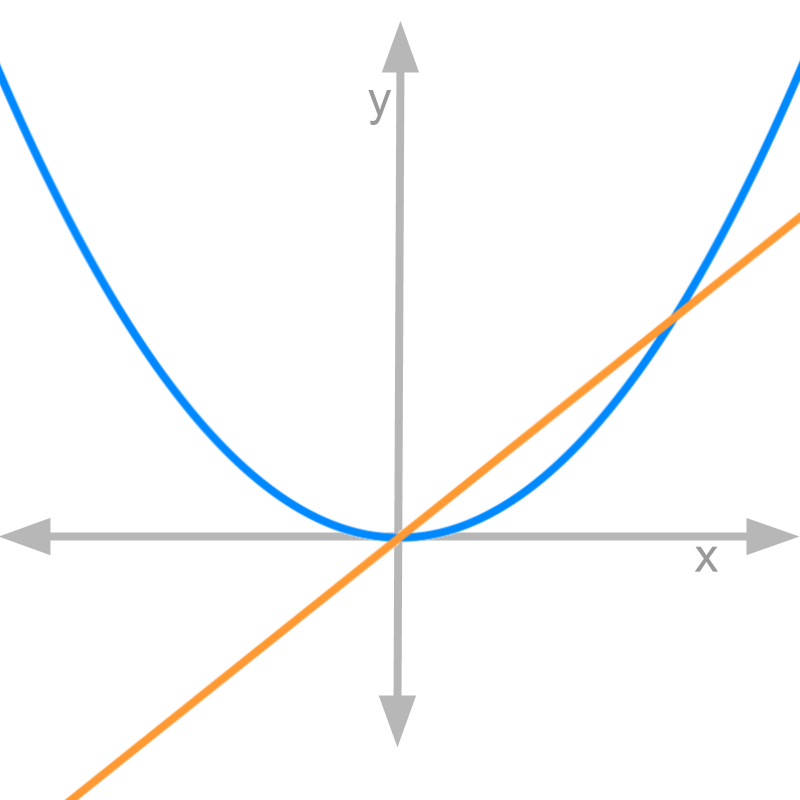Let us consider $y=\frac{{x}^{2}}{2}$$y = \frac{{x}^{2}}{2}$ and $\frac{dy}{dx}=x$$\frac{\mathrm{dy}}{\mathrm{dx}} = x$. The figure depicts both the functions. Blue color curve is $y=\frac{{x}^{2}}{2}$$y = \frac{{x}^{2}}{2}$ and orange color line is $\frac{dy}{dx}=x$$\frac{\mathrm{dy}}{\mathrm{dx}} = x$.
note: The plot is not to the scale on x and y axes.

The rate of change of blue curve is plotted as orange line.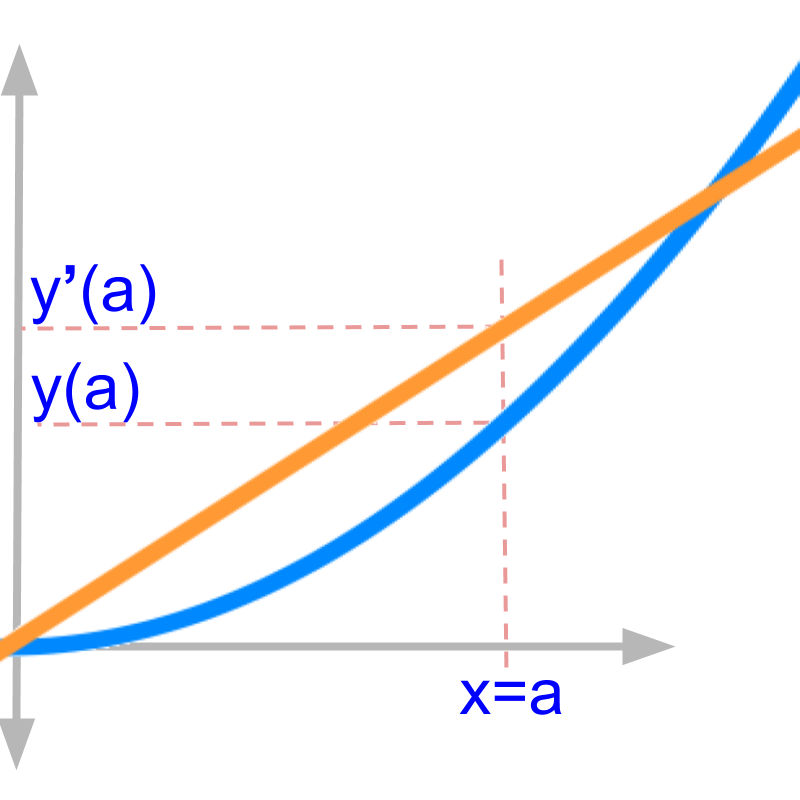The figure zooms in a small part of the plots.

•   $y\left(a\right)$$y \left(a\right)$ is the value function evaluates to at $x=a$$x = a$

•   $y\prime \left(a\right)$ is the rate of change of $y$$y$ at $x=a$$x = a$

This result is known from the algebraic derivations. Let us see what this means in the given curve.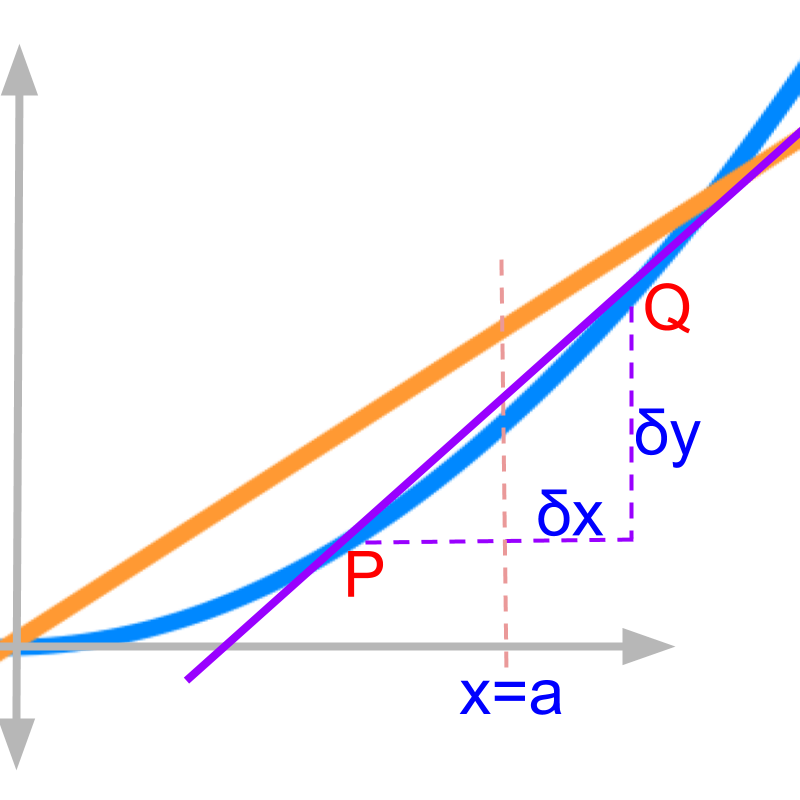Considering $y=\frac{{x}^{2}}{2}$$y = \frac{{x}^{2}}{2}$ and $\frac{dy}{dx}=x$$\frac{\mathrm{dy}}{\mathrm{dx}} = x$ given in the figure.

The derivative in first principles is given as
$y\prime \left(x\right)$
$=\frac{d}{dx}y$$= \frac{d}{\mathrm{dx}} y$
$=\underset{\delta x\to 0}{lim}\frac{\delta y}{\delta x}$$= {\lim}_{\delta x \to 0} \frac{\delta y}{\delta x}$

$\delta x$$\delta x$ at $x=a$$x = a$ is shown in the figure.
$\delta y$$\delta y$ for the $\delta x$$\delta x$ is shown in the figure.

the derivative is derived using limit $\delta x$$\delta x$ tending to $0$$0$

The figure shows non-zero $\delta x$$\delta x$ and corresponding $\delta y$$\delta y$ before limit is applied.

The points $P$$P$ and $Q$$Q$ are on the curve separated by $\delta x$$\delta x$ on $x=a$$x = a$. Consider the line passing through the points $P$$P$ and $Q$$Q$. The line is a secant as the line passes through two points on the curve.

It shows non-zero $\delta x$$\delta x$ and corresponding $\delta y$$\delta y$ before limit is applied. The slope of the line $\overline{PQ}$$\overline{P Q}$ is "$\frac{\delta y}{\delta x}$$\frac{\delta y}{\delta x}$". The line passing through the points $P\left({x}_{1},{y}_{1}\right)$$P \left({x}_{1} , {y}_{1}\right)$ and $Q\left({x}_{1}+\delta x,{y}_{1}+\delta y\right)$$Q \left({x}_{1} + \delta x , {y}_{1} + \delta y\right)$
Slope of the secant
$=\frac{{y}_{2}-{y}_{1}}{{x}_{2}-{x}_{1}}$$= \frac{{y}_{2} - {y}_{1}}{{x}_{2} - {x}_{1}}$
$=\frac{\delta y}{\delta x}$$= \frac{\delta y}{\delta x}$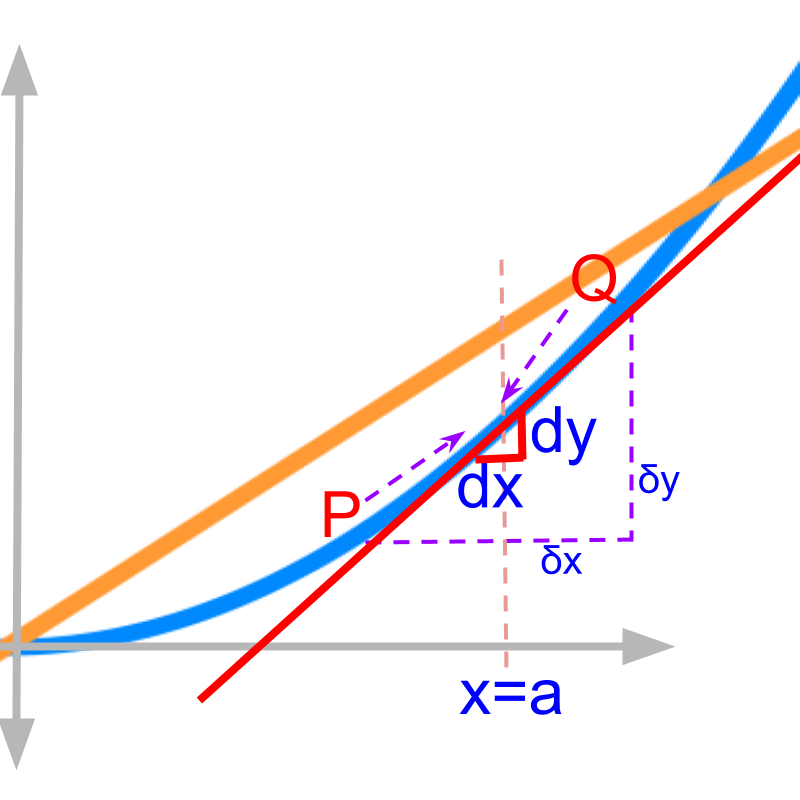Considering $y=\frac{{x}^{2}}{2}$$y = \frac{{x}^{2}}{2}$ and $\frac{dy}{dx}=x$$\frac{\mathrm{dy}}{\mathrm{dx}} = x$ given in the figure.

The derivative in first principles is given as
$y\prime \left(x\right)$
$=\underset{\delta x\to 0}{lim}\frac{\delta y}{\delta x}$$= {\lim}_{\delta x \to 0} \frac{\delta y}{\delta x}$

Applying the limit, the $\delta x$$\delta x$ and $\delta y$$\delta y$ reduces "close to $0$$0$", which is shown in red.
The points $P$$P$ and $Q$$Q$ at the two ends of $dx$$\mathrm{dx}$ moves towards each other. When limit $\delta x$$\delta x$ is close to zero, the two points $P$$P$ and $Q$$Q$ merge to become a single point. The points $P$$P$ and $Q$$Q$ move towards each other and merge.

The line passing through $P$$P$ and $Q$$Q$ is shown in red. As the limit is applied, the line touches the curve in only one point

The line is a "tangent", as the line touches at a single point on the curve.

The slope of the tangent at $x=a$$x = a$ is "$\frac{dy}{dx}{\mid }_{x=a}$$\frac{\mathrm{dy}}{\mathrm{dx}} {|}_{x = a}$". The rate of change is the slope at that point.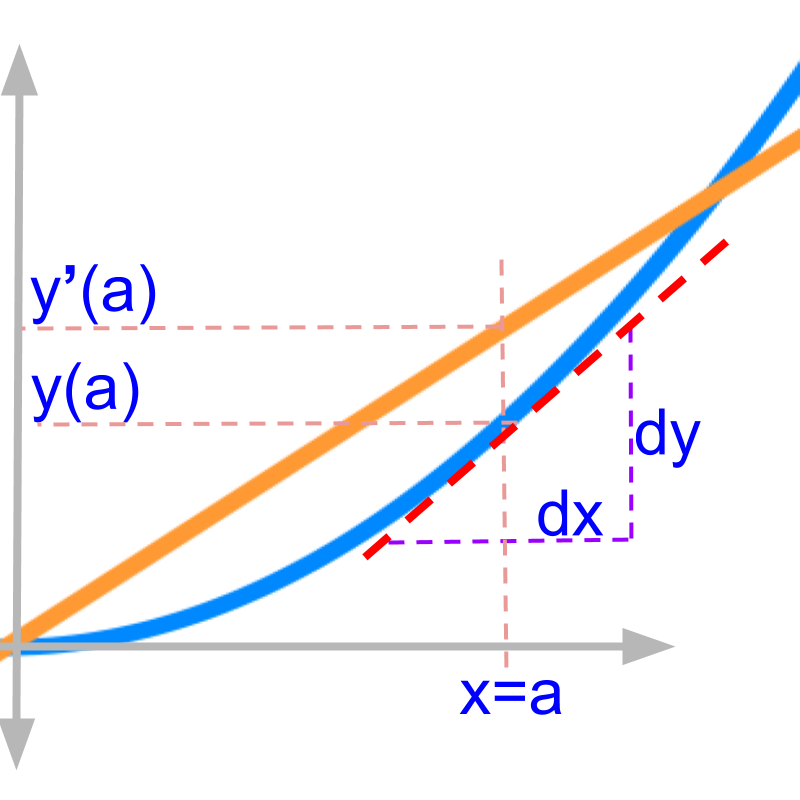$\frac{dy}{dx}{\mid }_{x=a}$$\frac{\mathrm{dy}}{\mathrm{dx}} {|}_{x = a}$ is the slope of the tangent on curve $y$$y$ at position $x=a$$x = a$. The tangent is shown in red dotted line.

analysing the curves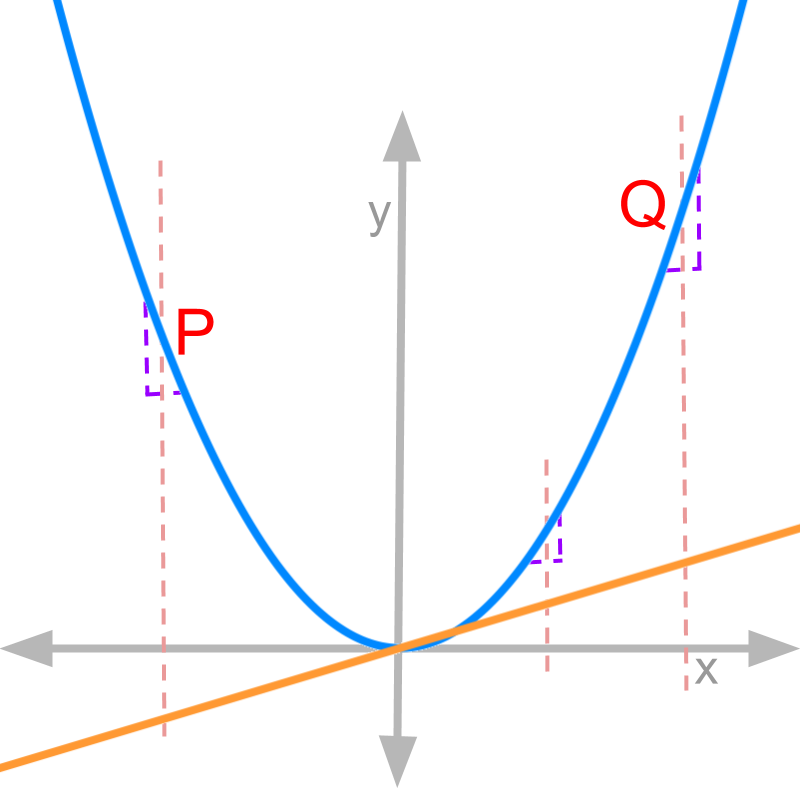For a function $f\left(x\right)$$f \left(x\right)$, the slope of tangent at $x=a$$x = a$ is $\frac{d}{dx}f\left(x\right){\mid }_{x=a}$$\frac{d}{\mathrm{dx}} f \left(x\right) {|}_{x = a}$.The figure shows $f\left(x\right)$$f \left(x\right)$ in blue and $\frac{d}{dx}f\left(x\right)$$\frac{d}{\mathrm{dx}} f \left(x\right)$ in orange. Three positions are identified with $3$$3$ vertical lines.

The slope of $f\left(x\right)$$f \left(x\right)$ at the position $P$$P$ is negative or decreasing rate of change. This is also evident from the negative value at the same position in orange line.

The slope of $f\left(x\right)$$f \left(x\right)$ at the position $Q$$Q$ is "positive or increasing rate of change". This is also evident from the positive value at the same position in orange line.

The slope of $f\left(x\right)$$f \left(x\right)$ at the position $x=0$$x = 0$ is "zero". This is also evident from the zero value at the same position in orange line.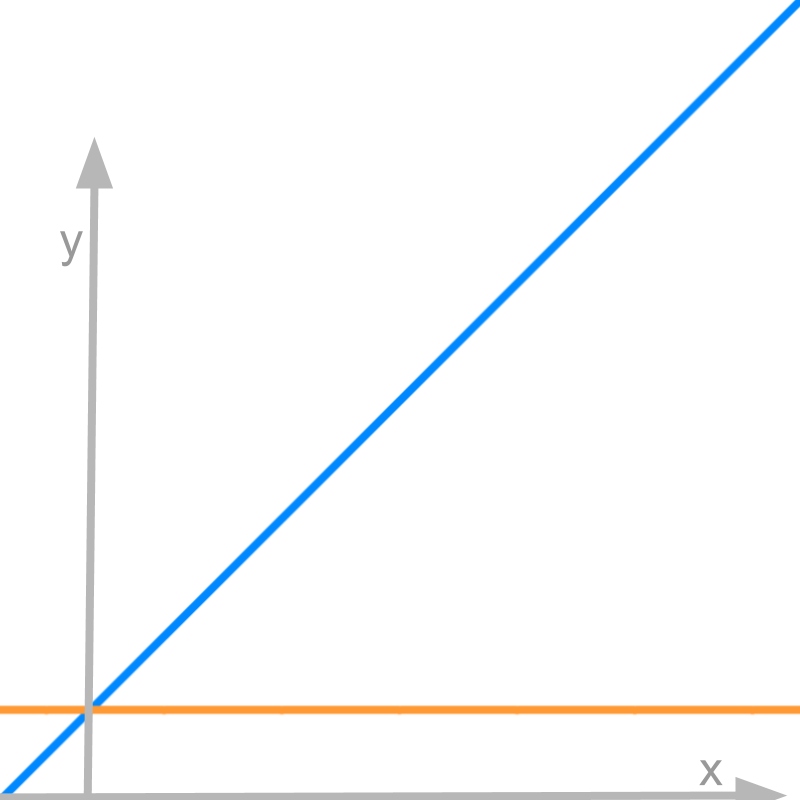Figure shows $y=x+1$$y = x + 1$ in blue and $\frac{dy}{dx}=1$$\frac{\mathrm{dy}}{\mathrm{dx}} = 1$ in orange. From the figure, we understand that "the function has constant rate of change". This is evident from the flat orange line.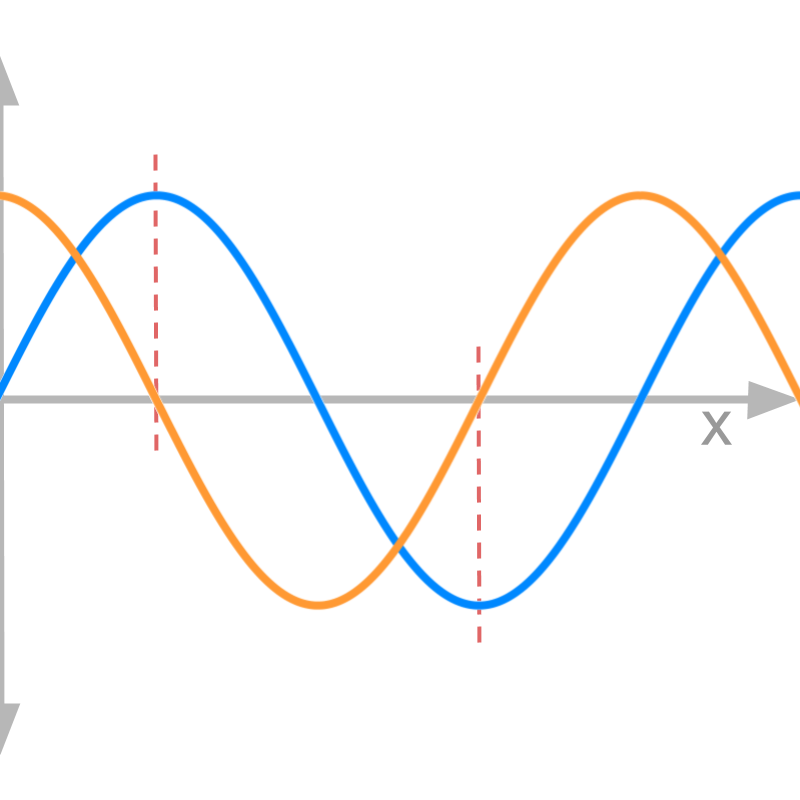The figure shows $y=\mathrm{sin}x$$y = \sin x$ in blue and $\frac{dy}{dx}=\mathrm{cos}x$$\frac{\mathrm{dy}}{\mathrm{dx}} = \cos x$ in orange.

•   when $y$$y$ reaches maximum, the rate of change is $0$$0$

•   when $y$$y$ reaches minimum, the rate of change is $0$$0$ This is evident from the values on orange line, the derivative of the function, having value 0 at the positions of maxima and minima.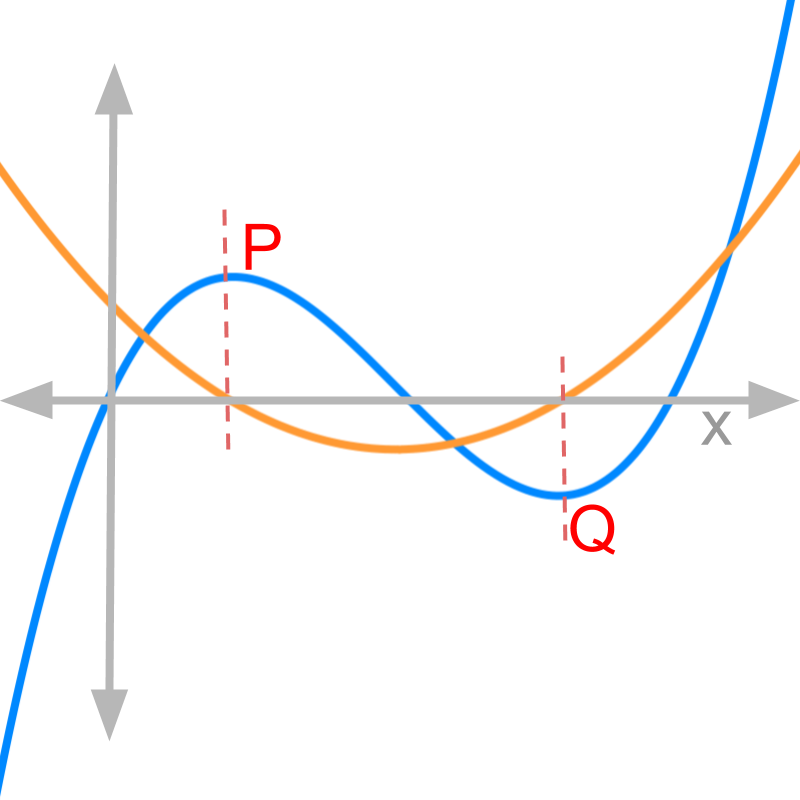The figure shows $y$$y$ in blue and $\frac{dy}{dx}$$\frac{\mathrm{dy}}{\mathrm{dx}}$ in orange. Two positions are identified.

•   when $y$$y$ reaches maximum, the rate of change crosses $0$$0$ from positive to negative

•   when $y$$y$ reaches minimum, the rate of change crosses $0$$0$ from negative to positive

This is evident from the values on orange line, the derivative of the function, crossing the x axis at the positions of maxima and minima.

The slope of the tangent on curve is the derivative evaluated at that point.

example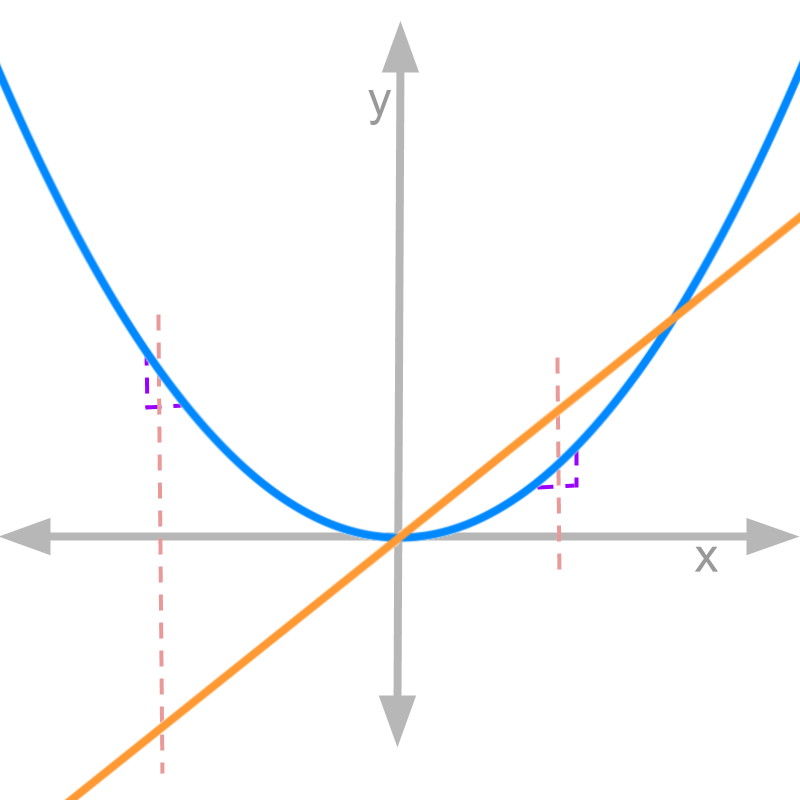The figure shows $y$$y$ in blue and $\frac{dy}{dx}$$\frac{\mathrm{dy}}{\mathrm{dx}}$ in orange. The observations are

•   for negative values of $x$$x$, the rate of change is negative

•   for positive values of $x$$x$, the rate of change is positive

•   at $x=0$$x = 0$, the rate of change crosses $0$$0$ from negative to positive

summaryGraphical Meaning of Derivative: For a function $f\left(x\right)$$f \left(x\right)$, the derivative $f\prime \left(x\right)$ is another function of the variable.

•  at a point $x=a$$x = a$, the rate of change of the curve is $f\prime \left(a\right)$

•  at a point $x=a$$x = a$, the slope of the tangent is $f\prime \left(a\right)$.

•  at the maxima of the curve $f\left({a}_{1}\right)$$f \left({a}_{1}\right)$, the derivative $f\prime \left({a}_{1}\right)$ crosses $0$$0$ from positive rate of change to negative rate of change.

•  at the minima of the curve $f\left({a}_{2}\right)$$f \left({a}_{2}\right)$, the derivative $f\prime \left({a}_{2}\right)$ crosses $0$$0$ from negative rate of change to positive rate of change.

Outline

The outline of material to learn "Differential Calculus" is as follows.

•   Detailed outline of Differential Calculus

→   Application Scenario

→   Differentiation in First Principles

→   Graphical Meaning of Differentiation

→   Differntiability

→   Algebra of Derivatives

→   Standard Results